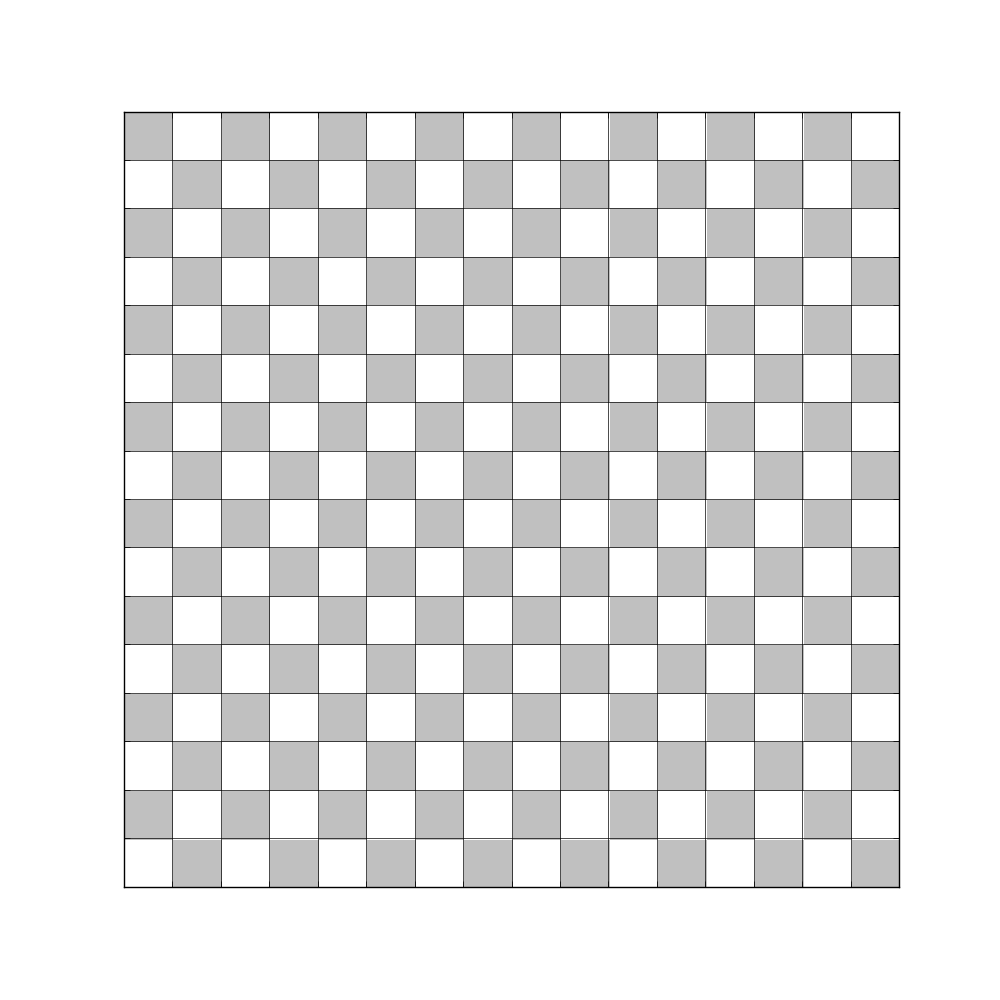# Misalignment imshow vs. grid lines

Hi,

I tried to plot a grid over a small image (24x24) and some grid lines are mis-aligned, can anybody confirm the behavior ?

Here is a sample script:

import numpy as np
import matplotlib.pyplot as plt

n = 16
fig = plt.figure(figsize=(10,10))
Z = np.array(([0,1](n//2) + [1,0](n//2))*(n//2)).reshape(n,n)
plt.imshow(Z, interpolation=‘nearest’, cmap=plt.cm.gray, extent=[0,n,0,n], alpha=.25)
plt.xticks(np.arange(0,n), [])
plt.yticks(np.arange(0,n), [])
plt.grid(ls=‘solid’)
plt.show()

Here is a sample output (it’s worse on screen):Nicolas